Next: Macrostates and microstates Up: Heat and work Previous: Heat and work

## A brief history of heat and work

In 1789 the French scientist Antoine Lavoisier published a famous treatise on Chemistry which, amongst other things, demolished the then prevalent theory of combustion. This theory, known to history as the phlogiston theory, is so extraordinary stupid that it is not even worth describing. In place of phlogiston theory, Lavoisier proposed the first reasonably sensible scientific interpretation of heat. Lavoisier pictured heat as an invisible, tasteless, odourless, weightless fluid, which he called calorific fluid. He postulated that hot bodies contain more of this fluid than cold bodies. Furthermore, he suggested that the constituent particles of calorific fluid repel one another, causing heat to flow from hot to cold bodies when they are placed in thermal contact.

The modern interpretation of heat is, or course, somewhat different to Lavoisier's calorific theory. Nevertheless, there is an important subset of problems involving heat flow for which Lavoisier's approach is rather useful. These problems often crop up as examination questions. For example: A clean dry copper calorimeter contains 100 grams of water at 30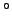degrees centigrade. A 10 gram block of copper heated to 60centigrade is added. What is the final temperature of the mixture?''. How do we approach this type of problem? Well, according to Lavoisier's theory, there is an analogy between heat flow and incompressible fluid flow under gravity. The same volume of liquid added to containers of different cross-sectional area fills them to different heights. If the volume is, and the cross-sectional area is, then the height is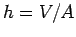. In a similar manner, the same quantity of heat added to different bodies causes them to rise to different temperatures. If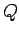is the heat andis the (absolute) temperature then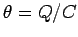, where the constant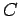is termed the heat capacity. [This is a somewhat oversimplified example. In general, the heat capacity is a function of temperature, so that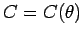.] Now, if two containers filled to different heights with a free flowing incompressible fluid are connected together at the bottom, via a small pipe, then fluid will flow under gravity, from one to the other, until the two heights are the same. The final height is easily calculated by equating the total fluid volume in the initial and final states. Thus,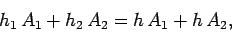(114)

giving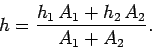(115)

Here,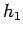and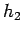are the initial heights in the two containers,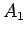and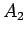are the corresponding cross-sectional areas, and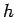is the final height. Likewise, if two bodies, initially at different temperatures, are brought into thermal contact then heat will flow, from one to the other, until the two temperatures are the same. The final temperature is calculated by equating the total heat in the initial and final states. Thus,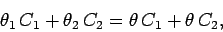(116)

giving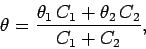(117)

where the meaning of the various symbols should be self-evident.

The analogy between heat flow and fluid flow works because in Lavoisier's theory heat is a conserved quantity, just like the volume of an incompressible fluid. In fact, Lavoisier postulated that heat was an element. Note that atoms were thought to be indestructible before nuclear reactions were discovered, so the total amount of each element in the Universe was assumed to be a constant. If Lavoisier had cared to formulate a law of thermodynamics from his calorific theory then he would have said that the total amount of heat in the Universe was a constant.

In 1798 Benjamin Thompson, an Englishman who spent his early years in pre-revolutionary America, was minister for war and police in the German state of Bavaria. One of his jobs was to oversee the boring of cannons in the state arsenal. Thompson was struck by the enormous, and seemingly inexhaustible, amount of heat generated in this process. He simply could not understand where all this heat was coming from. According to Lavoisier's calorific theory, the heat must flow into the cannon from its immediate surroundings, which should, therefore, become colder. The flow should also eventually cease when all of the available heat has been extracted. In fact, Thompson observed that the surroundings of the cannon got hotter, not colder, and that the heating process continued unabated as long as the boring machine was operating. Thompson postulated that some of the mechanical work done on the cannon by the boring machine was being converted into heat. At the time, this was quite a revolutionary concept, and most people were not ready to accept it. This is somewhat surprising, since by the end of the eighteenth century the conversion of heat into work, by steam engines, was quite commonplace. Nevertheless, the conversion of work into heat did not gain broad acceptance until 1849, when an English physicist called James Prescott Joule published the results of a long and painstaking series of experiments. Joule confirmed that work could indeed be converted into heat. Moreover, he found that the same amount of work always generates the same quantity of heat. This is true regardless of the nature of the work (e.g., mechanical, electrical, etc.). Joule was able to formulate what became known as the work equivalent of heat. Namely, that 1 newton meter of work is equivalent to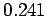calories of heat. A calorie is the amount of heat required to raise the temperature of 1 gram of water by 1 degree centigrade. Nowadays, we measure both heat and work in the same units, so that one newton meter, or joule, of work is equivalent to one joule of heat.

In 1850, the German physicist Clausius correctly postulated that the essential conserved quantity is neither heat nor work, but some combination of the two which quickly became known as energy, from the Greek energia meaning in work.'' According to Clausius, the change in the internal energy of a macroscopic body can be written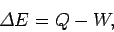(118)

whereis the heat absorbed from the surroundings, and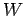is the work done on the surroundings. This relation is known as the first law of thermodynamics.Next: Macrostates and microstates Up: Heat and work Previous: Heat and work
Richard Fitzpatrick 2006-02-02